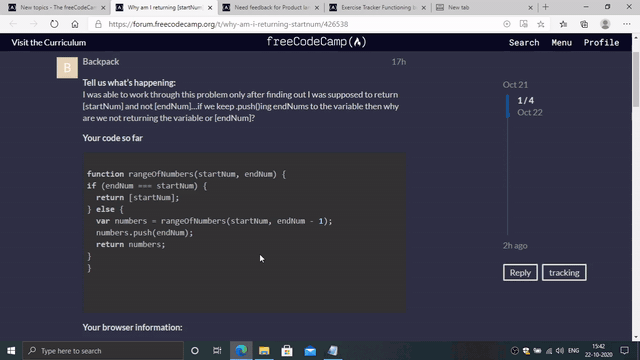# Why am I returning [startNum]

Tell us what’s happening:
I was able to work through this problem only after finding out I was supposed to return [startNum] and not [endNum]…if we keep .push()ing endNums to the variable then why are we not returning the variable or [endNum]?

``````
function rangeOfNumbers(startNum, endNum) {
if (endNum === startNum) {
return [startNum];
} else {
var numbers = rangeOfNumbers(startNum, endNum - 1);
numbers.push(endNum);
return numbers;
}
}

``````

User Agent is: `Mozilla/5.0 (Macintosh; Intel Mac OS X 10_15_7) AppleWebKit/537.36 (KHTML, like Gecko) Chrome/86.0.4240.80 Safari/537.36`.

Challenge: Use Recursion to Create a Range of Numbers

Because `[startNum]` is only returned `startNum === endNum`, that means it is exactly the same result as `[endNum]`.

1 Like

I guess my question then is how after we reach the base case, how do all the endNums make it into the same array as the starNum.

With this line:

``````numbers.push(endNum);
``````

This video might help you understand the code.Let me walk through what happens in this function with a short example: `rangeOfNumbers(1,3)`

• is 1 equal to 3? No.
• call `rangeOfNumbers(1,2)`. The next line can’t execute until `rangeOfNumbers(1,2)` returns, so let’s look at what happens in `rangeOfNumbers(1,2)`:
• is 1 equal to 2? No.
• call `rangeOfNumbers(1,1)`. The next line can’t execute until `rangeOfNumbers(1,1)` returns, so let’s look at what happens in `rangeOfNumbers(1,1)`:
• is 1 equal to 1? Yes.
• return ``
• Now that `rangeOfNumbers(1,1)` has returned, `numbers` in the `rangeOfNumbers(1,2)`is equal to``
• Push `2` onto `numbers`.
• return `[1, 2]`
• Now that `rangeOfNumbers(1,2)` has returned, `numbers` in `rangeOfNumbers(1,3)` is equal to `[1, 2]`.
• push `3` onto `numbers`
• return `[1, 2, 3]`
1 Like